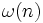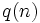# Prime divisor count function

This article defines an arithmetic function or number-theoretic function: a function from the natural numbers to a ring (usually, the ring of integers, rational numbers, real numbers, or complex numbers).
View a complete list of arithmetic functions

## Definition

Letbe a natural number. The prime divisor count function of, denoted, is defined as the number of prime divisors of.

## Relation with other arithmetic functions

• Mobius function: This is defined asfora square-free number, and isotherwise.
• Divisor count function: This is denoted, and is defined as the total number of positive divisors of.
• Largest prime power divisor: Denoted, this is defined as the largest prime power dividing. We have:.